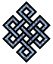fail2ban bad ip database: ip 183.196.172.36

| ip database | live view | stats | report | help | api key:

 ip: 183.196.172.36 hostname: 183.196.172.36 country:[CN] China first reported: 21.06.2018 23:45.01 GMT+0200 last reported: 29.01.2019 00:55.04 GMT+0200 time period: 221d 02h 10m 03s total reports: 3 reported by: 1 host(s) filter(s): ssh (3) tor exit node no badips.com db Lookupport scan of '183.196.172.36':

[-hide]
```# Nmap 6.40 scan initiated Thu Jun 21 23:45:03 2018 as: /usr/bin/nmap -sU -sS -O 183.196.172.36
Nmap scan report for 183.196.172.36
Host is up (0.23s latency).
Not shown: 999 open|filtered ports, 971 closed ports
PORT      STATE    SERVICE
21/tcp    filtered ftp
22/tcp    open     ssh
23/tcp    open     telnet
25/tcp    filtered smtp
30/tcp    open     unknown
80/tcp    filtered http
81/tcp    filtered hosts2-ns
82/tcp    filtered xfer
88/tcp    filtered kerberos-sec
90/tcp    filtered dnsix
99/tcp    open     metagram
110/tcp   filtered pop3
135/tcp   filtered msrpc
139/tcp   filtered netbios-ssn
443/tcp   filtered https
445/tcp   filtered microsoft-ds
801/tcp   filtered device
1433/tcp  filtered ms-sql-s
1521/tcp  filtered oracle
3306/tcp  filtered mysql
3389/tcp  filtered ms-wbt-server
8000/tcp  open     http-alt
8010/tcp  filtered xmpp
8011/tcp  filtered unknown
8080/tcp  filtered http-proxy
8081/tcp  filtered blackice-icecap
9000/tcp  open     cslistener
10010/tcp open     rxapi
10012/tcp open     unknown
500/udp   open     isakmp
No exact OS matches for host (If you know what OS is running on it, see http://nmap.org/submit/ ).
TCP/IP fingerprint:
OS:SCAN(V=6.40%E=4%D=6/21%OT=22%CT=1%CU=%PV=N%G=Y%TM=5B2C1E49%P=x86_64-pc-l
OS:inux-gnu)SEQ(SP=107%GCD=1%ISR=108%TI=I%CI=I%TS=A)OPS(O1=M5B4NW3ST11%O2=M
OS:578NW3ST11%O3=M280NW3NNT11%O4=M5B4NW3ST11%O5=M218NW3ST11%O6=M109ST11)WIN
OS:(W1=FC00%W2=FC00%W3=FC00%W4=FC00%W5=FC00%W6=FC00)ECN(R=Y%DF=N%TG=FF%W=FC
OS:00%O=M5B4NW3SLL%CC=N%Q=)T1(R=Y%DF=N%TG=FF%S=O%A=S+%F=AS%RD=0%Q=)T2(R=N)T
OS:3(R=N)T4(R=Y%DF=N%TG=FF%W=0%S=A%A=Z%F=R%O=%RD=0%Q=)T5(R=Y%DF=N%TG=FF%W=0
OS:%S=Z%A=S+%F=AR%O=%RD=0%Q=)T6(R=Y%DF=N%TG=FF%W=0%S=A%A=Z%F=R%O=%RD=0%Q=)T
OS:7(R=N)U1(R=N)IE(R=Y%DFI=S%TG=FF%CD=S)

OS detection performed. Please report any incorrect results at http://nmap.org/submit/ .
# Nmap done at Thu Jun 21 23:53:13 2018 -- 1 IP address (1 host up) scanned in 492.09 seconds
```
```Σ = 68 | Δt = 0.0036978721618652s
```